## What is Joint Probability Density Function or Joint PDF?

Joint PDF is simply the PDF of two or more random variables.
The joint probability density function of any two random variables X and Y can be defined as the partial derivative of the joint cumulative distribution function, with respect to dummy variables x and y.
Mathematically-

## Watch the Complete Video Here

Probability Density Function (PDF) - Definition, Basics and Properties of Probability Density Function (PDF) with Derivation and Proof

## Joint PDF Formula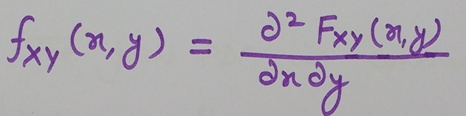Joint PDF Formula

Now we will discuss the properties of joint probability density function (joint PDF)

Property 1- Joint PDF is non-negative.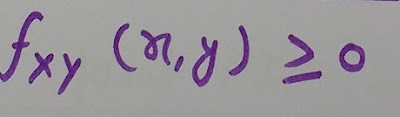Joint PDF Property

Since joint PDF is a derivative of joint cumulative distribution function (Joint CDF), which is also a non negative function.
Therefore joint PDF is always positive.

Cumulative Distribution Function (CDF) - Properties of CDF - CDF Definition, Basics - Continuous and Discrete CDF

Property 2- The joint PDF is continuous everywhere as the joint CDF is continuous and we know that it is the derivative of joint CDF.

Property 3- The total volume under the surface of joint PDF is equal to Unity.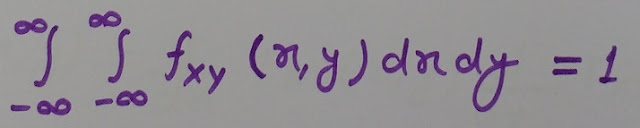Joint PDF Property

Now we will discuss an important property of two statistically independent random variables X and Y.

## Statistically Independent Random Variables X and Y

For two statistically independent random variables X and Y-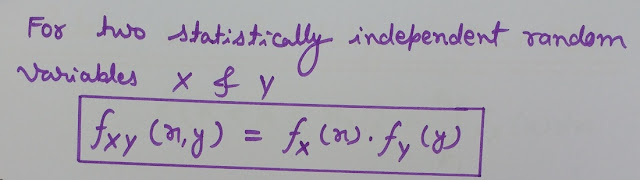Statistically independent random variables X and Y

Joint Cumulative Distribution Function - Joint Distribution Function - Combined CDF

Proof-Statistically Independent Random Variables X and YRelation between Probability and Joint PDF

On extending this relation to two random variables X and Y-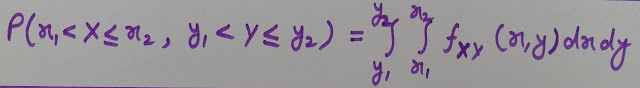Relation between Probability and Joint PDF for two Random Variables

Conditional Probability Density Function (Conditional PDF) - Properties of Conditional PDF with Derivation

## Relationship between joint PDF and Probability for statistically independent random variables X and Y

If two random variables X and Y are statistically independent, then the joint PDF of X and Y is given as the product of two separate PDFs.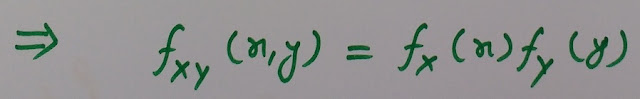Relationship between joint PDF and Probability for statistically independent random variables X and Y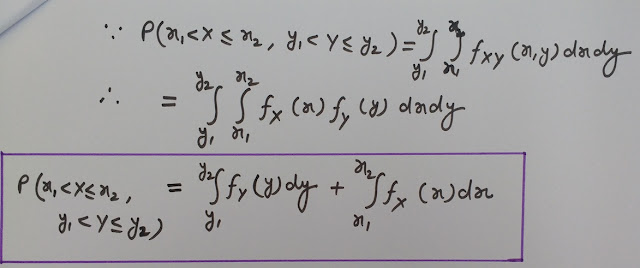Relationship between joint PDF and Probability for statistically independent random variables  X and Y